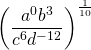# 9.7 Rational Exponents (Increased Difficulty)

Simplifying rational exponents equations that are more difficult generally involves two steps. First, reduce inside the brackets. Second, multiplu the power outside the brackets for all terms inside.

Example 9.7.1

Simplify the following rational exponent expression: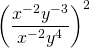First, simplifying inside the brackets gives: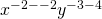Or: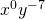Which simplifies to: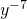Second, taking the exponent 2 outside the brackets and applying it to the reduced expression gives: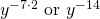Therefore:Example 9.7.2

Simplify the following rational exponent expression: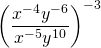First, simplifying inside the brackets gives: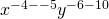Or: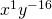Which simplifies to: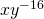Second, taking the exponent −3 outside the brackets and applying it to the reduced expression gives: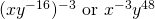Therefore: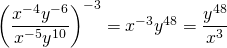Example 9.7.3

Simplify the following rational exponent expression: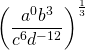First, simplifying inside the brackets gives: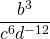Second, taking the exponentoutside the brackets and applying it to the reduced expression gives: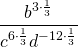Or: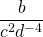Which simplifies to: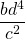# Questions

Simplify the following rational exponents.

1.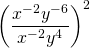2.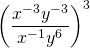3.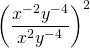4.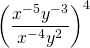5.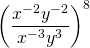6.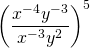7.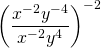8.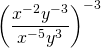9.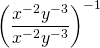10.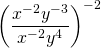11.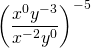12.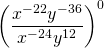13.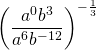14.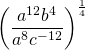15.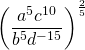16.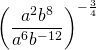17.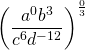18.